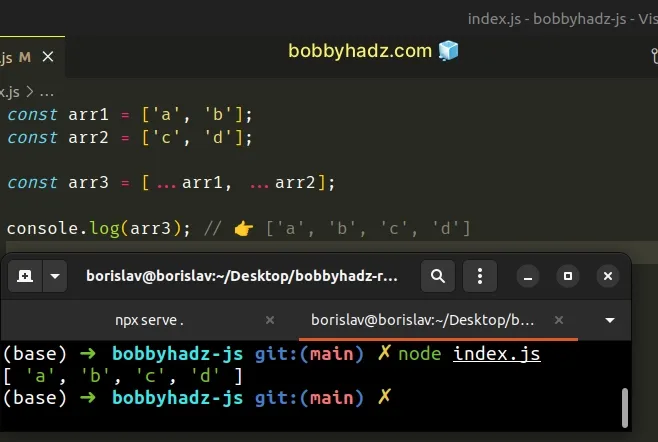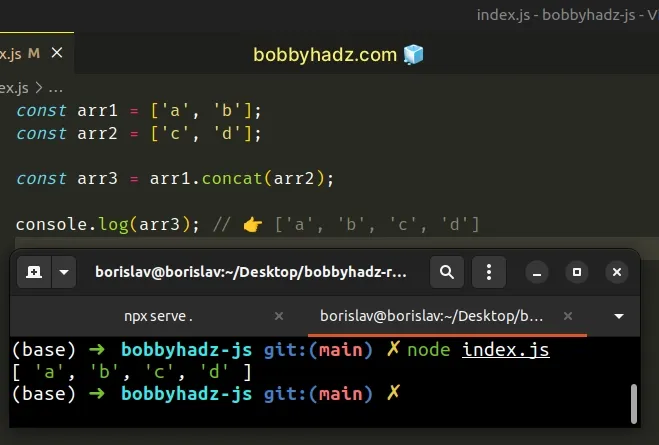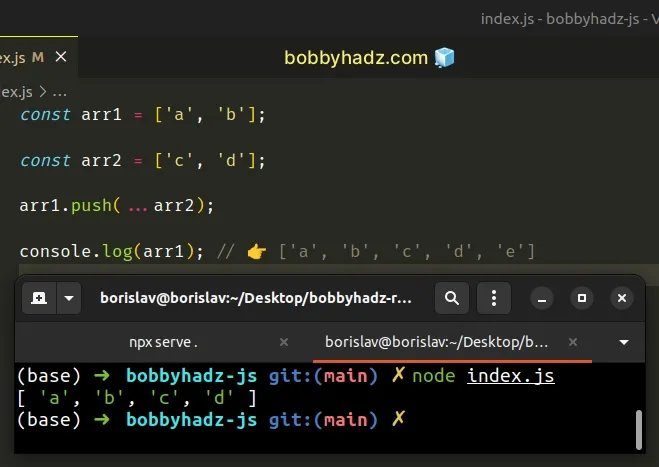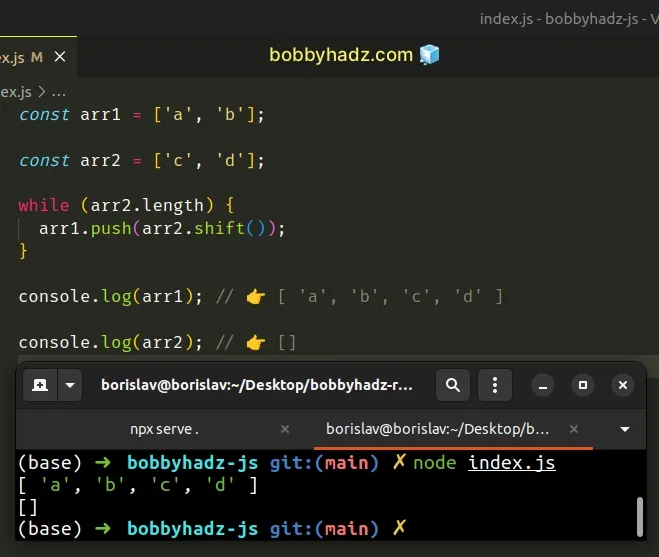# How to Append one Array to Another in JavaScriptLast updated: Dec 26, 2022
5 min## #Append one Array to another Array in JavaScript

To append one array to another:

1. Use the spread syntax (...) to unpack the values of the two arrays into a third array.
2. The spread syntax will return a new array by appending the second array to the first array.
index.js
```Copied!```const arr1 = ['a', 'b'];
const arr2 = ['c', 'd'];

const arr3 = [...arr1, ...arr2];

console.log(arr3); // 👉️ ['a', 'b', 'c', 'd']
``````We used the spread syntax (...) to unpack the values of two arrays into a third array.

The easiest way to think about it is:

1. We grab all the values of `arr1` and add them to `arr3`.
2. We grab all the values of `arr2` and add them to `arr3`.
The spread syntax doesn't change the contents of `arr1` and `arr2`.

The order in which we unpacked the arrays is preserved and all of the elements of `arr1` come before the elements of `arr2`.

The spread syntax (...) is commonly used when you need to add new elements to an array without changing the contents of the original array.

index.js
```Copied!```const arr1 = ['a', 'b'];

const arr2 = [...arr1, 'c', 'd'];

console.log(arr2); // 👉️ ['a', 'b', 'c', 'd']
``````

Using the spread syntax to append one another to another is very performant.

## #Append one Array to another array using `Array.concat()`

Alternatively, you can use the `Array.concat()` method.

The `Array.concat()` method will merge the two arrays into a third array and will return the result.

index.js
```Copied!```const arr1 = ['a', 'b'];
const arr2 = ['c', 'd'];

const arr3 = arr1.concat(arr2);

console.log(arr3); // 👉️ ['a', 'b', 'c', 'd']
``````We used the Array.concat() method to merge two arrays into a third.

The method doesn't change the contents of the original arrays, instead it returns a new array.

The method takes the array(s) you want to concatenate into the new array as parameters.

Here's an example of calling the `concat()` method with multiple arrays.

index.js
```Copied!```const arr1 = ['a', 'b'];

const arr2 = arr1.concat(['c'], ['d'], ['e']);

console.log(arr2); // 👉️ ['a', 'b', 'c', 'd', 'e']
``````

All of the arrays we passed to the `Array.concat()` method, including `arr1` got merged into a new array.

You can even pass non-array values to the `concat()` method.

index.js
```Copied!```const arr1 = ['a', 'b'];

const arr2 = arr1.concat('c', 'd', ['e']);

console.log(arr2); // 👉️ ['a', 'b', 'c', 'd', 'e']
``````

We passed two strings and an array to the `concat()` method and they all got merged into the new array.

## #Append one Array to another Array using `push()`

An alternative approach is to use the `push()` method.

The `Array.push()` method is used to add one or more elements to the end of an array.

index.js
```Copied!```const arr1 = ['a', 'b'];

const arr2 = ['c', 'd'];

arr1.push(...arr2);

console.log(arr1); // 👉️ ['a', 'b', 'c', 'd', 'e']
``````We used the spread operator (...) to unpack the values of `arr2` when calling the Array.push method.

The `push()` method takes one or more values as parameters. The parameters get pushed to the end of the array.

Note that the `Array.push()` method changes the contents of the array on which it was called.

The method mutates the array in place.

You can also use a simple `while` loop.

## #Append one Array to another Array using a `while` loop

This is a three-step process:

1. Use a `while` loop to iterate over the second array.
2. On each iteration, use the `Array.shift()` method to remove the first element from the array.
3. Use the `Array.push()` method to push the removed element into the other array.
index.js
```Copied!```const arr1 = ['a', 'b'];

const arr2 = ['c', 'd'];

while (arr2.length) {
arr1.push(arr2.shift());
}

console.log(arr1); // 👉️ [ 'a', 'b', 'c', 'd' ]

console.log(arr2); // 👉️ []
``````We used a `while` loop to iterate for as long as the length of `arr2` is greater than `0`.

On each iteration, we use the `Array.shift()` method to remove and return the first element from the second array.

index.js
```Copied!```const arr2 = ['c', 'd'];

console.log(arr2.shift()); // 👉️ c
``````

We directly passed the output of the `Array.shift()` method to the `Array.push()` method to push the element into the first array.

The `while` loop appends the contents of the second array to the first array, in place.

You can also use the `Array.forEach()` method.

## #Append one Array to another Array using `Array.forEach()`

This is a two-step process:

1. Use the `Array.forEach()` method to iterate over the second array.
2. Use the `Array.push()` method to push each element into the first array.
index.js
```Copied!```const arr1 = ['a', 'b'];

const arr2 = ['c', 'd'];

for (const element of arr2) {
arr1.push(element);
}

console.log(arr1); // 👉️ [ 'a', 'b', 'c', 'd' ]
``````

The function we passed to the Array.forEach method gets called with each element in the array.

On each iteration, we use the `Array.push()` method to push the element into the first array.

This approach mutates the contents of the first array only, whereas the `while` loop approach changed both arrays in place.

## #Append one Array to another Array using `for...of`

You can also use a `for...of` loop to append one array to another array.

index.js
```Copied!```const arr1 = ['a', 'b'];

const arr2 = ['c', 'd'];

for (const element of arr2) {
arr1.push(element);
}

console.log(arr1); // 👉️ [ 'a', 'b', 'c', 'd' ]
``````

The for...of statement is used to loop over iterable objects like arrays, strings, `Map`, `Set` and `NodeList` objects and `generators`.

On each iteration, we use the `Array.push()` method to add the element of the second array to the first array.

## #Append one Array to another Array using a `for` loop

You can also use a basic `for` loop to append one array to another array.

index.js
```Copied!```const arr1 = ['a', 'b'];

const arr2 = ['c', 'd'];

for (let index = 0; index < arr2.length; index++) {
arr1.push(arr2[index]);
}

console.log(arr1); // 👉️ [ 'a', 'b', 'c', 'd' ]
``````

The syntax for a basic `for` loop is quite verbose and we have to make use of the index to access the array.

Which approach you pick is a matter of personal preference. I'd use the spread syntax because it's quite performant and easy to read.

index.js
```Copied!```const arr1 = ['a', 'b'];
const arr2 = ['c', 'd'];

const arr3 = [...arr1, ...arr2];

console.log(arr3); // 👉️ ['a', 'b', 'c', 'd']
``````